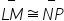Mathematics
Easy

Question

# What is the justification?If LM = NP, then## Definition of congruenceReflexive Property of CongruenceSegment Addition PostulateDefinition of MidpointHint:

## The correct answer is: Definition of congruence

### Here , we have to find the reason of (LM) ̅ ≅ (NP) ̅ Firstly, given is LM = NP , Now, (LM) ̅ ≅ (NP) ̅ , If lines are congruent then we use ≅ this symbol, And also both line are equal LM = NP which is given.Therefore, the reason of (LM) ̅ ≅ (NP) ̅ is Reflexive Property of congruence.The correct answer is Reflexive property of congruence.

In this question, we have to find the reason of (LM) ̅ ≅ (NP) ̅ . If the line are congruent then we also give then a sign of approximately equal ≅. Both lines are also equal that’s why its follow the Reflexive property of congruence.

### Related Questions to study#### With Turito Foundation.#### Get an Expert Advice From Turito.